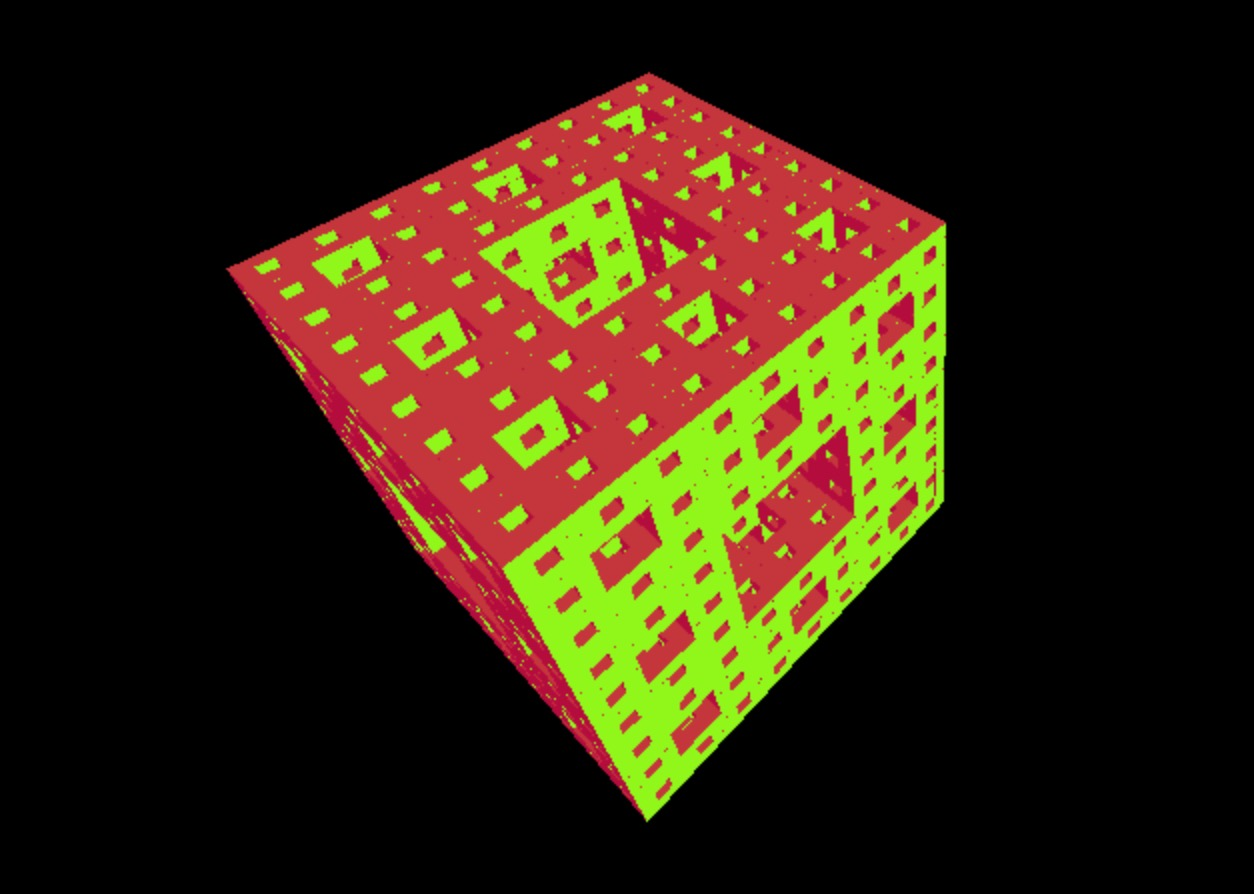Created: 6 Oct 2016, last rebuilt: 25 May 2017

# Menger sponge

I was inspired by FractalLab to try and make my own Menger Sponge animation.

My first attempt was in Three.js, by assembling the three-iteration sponge from smaller cubes. Pretty simple, but it got very slow very fast (at n=3 it’s basically unusable). See here for the result.It became obvious that this should be done with a shader. Many great tutorials exist to kick it off. I went through multiple iterations, starting with much worse performance than three.js:

• assembling the cube from “atoms”: slow (~1 fps for 3 iterations)
• knocking out empty spaces in all dimensions: slow (~1 fps for 3 iterations)
• using a repeating pattern (mod) to knock out empty spaces

The last one shows promise, but I’m sure it can be optimized much further. A project for another rainy day :)

Below’s the basic GLSL code for the last one

``````float basic_box(vec3 pos, vec3 b){
vec3 d = abs(pos) - b;
return min(max(d.x,max(d.y,d.z)),0.0) + length(max(d,0.0));
}

float map_menger5(vec3 p){

float main_width_b = 4.0;
float inf = 50.0;

float hole_x, hole_y, hole_z;
float hole_width_b = main_width_b / 3.0;

float menger = basic_box(p, vec3(main_width_b));

for (int iter=0; iter<5; iter++){

float hole_distance = hole_width_b * 6.0;

vec3 c = vec3(hole_distance);
vec3 q = mod(p + vec3(hole_width_b), c) - vec3(hole_width_b);

hole_x = basic_box(q, vec3(inf, hole_width_b, hole_width_b));
hole_y = basic_box(q, vec3(hole_width_b, inf, hole_width_b));
hole_z = basic_box(q, vec3(hole_width_b, hole_width_b, inf));

hole_width_b = hole_width_b / 3.0;        // reduce hole size for next iter
menger = max(max(max(menger, -hole_x), -hole_y), -hole_z); // subtract

}
return menger;
}

float trace(vec3 origin, vec3 ray){

float t = 0.0;
for (int i=0; i<32; ++i){
vec3 p = origin + ray * t;
float d = map_menger5(p);
t += d;
}
return t;
}

mat2 rotate(float theta){
return mat2(cos(theta), -sin(theta), sin(theta), cos(theta));
}

void mainImage( out vec4 fragColor, in vec2 fragCoord ) {

vec2 uv = fragCoord.xy / iResolution.xy;  // normalize coords
uv = uv * 2.0 - 1.0;                      // convert coords from 0,1 to -1,1
uv.x *= iResolution.x/iResolution.y;      // fix aspect

vec3 ray = normalize(vec3(uv.x, uv.y, 1.0));
vec3 origin = vec3(0.0,0.0,-4.0 -5.2*sin(iGlobalTime * 0.20));

float theta;
theta = iGlobalTime / 5.0;
ray.yz *= rotate(theta);
ray.xy *= rotate(theta);
origin.yz *= rotate(theta);
origin.xy *= rotate(theta);

float t = trace(origin, ray);
float fog = 1.0 / (1.0 + t * t * 0.05);
vec3 fc = vec3(fog) * vec3(0.8+0.2*sin(iGlobalTime * 0.1), 0.8+0.2*sin(iGlobalTime * 1.0),0.9+0.1*cos(iGlobalTime*1.0));
fragColor = vec4(fc,1.0);
}
``````